# NCERT Solutions class 7 Maths Chapter-8 Exercise 8.3

NCERT Solutions Class-7 Maths chapter-8 Comparing Quantities  Exercise-8.3 is prepared by academic team of pw all the questions of NCERT text book are solved step by step with proper and detail solutions explaining each and every questions . For More and additional questions of CBSE class 7 maths you can go to class 7 maths sections. NCERT class 7 Maths Solutions is the best way to enhanced your mathematics skill. And pw practice worksheet & question bank will help you a lot .

## NCERT Solutions class 7 Maths Chapter-8 Comparing Quantities

### Solutions of Chapter Comparing Quantities  Exercise-8.3

Question 1:
Tell what is the profit or loss in the following transactions.  Also find profit per cent or loss per cent in each case
(a) Gardening shears bought for Rs. 250 and sold for Rs. 325.
(b) A refrigerator bought for Rs. 12,000 and sold at Rs. 13,500.
(c) A cupboard bought for Rs. 2,500 and sold at Rs. 3,000.
(d) A skirt bought for Rs. 250 and sold at Rs. 150.

(a) It is given in the question that,
Cost price of the product = Rs 250
Also,
Selling price of the product = Rs 325
Therefore,
Profit = Selling Price – Cost Price
= 325 – 250
= Rs 75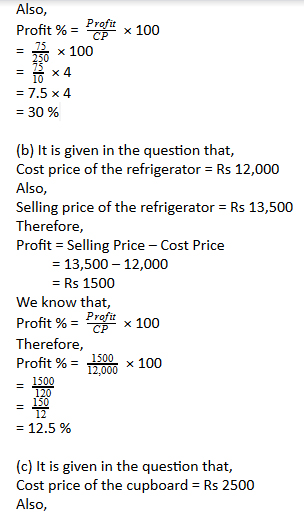Selling price of the refrigerator = Rs 13,500

Therefore,

Profit = Selling Price – Cost Price

= 13,500 – 12,000

= Rs 1500

We know that,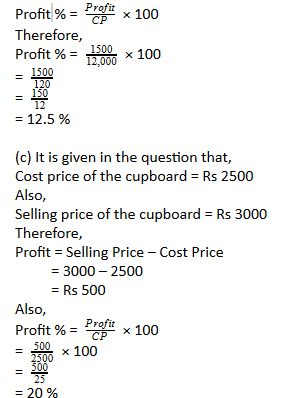(d) It is given in the question that,

Cost price of the skirt = Rs 250

Also,

Selling price of the skirt = Rs 150

Therefore,

Loss = Cost Price – Selling Price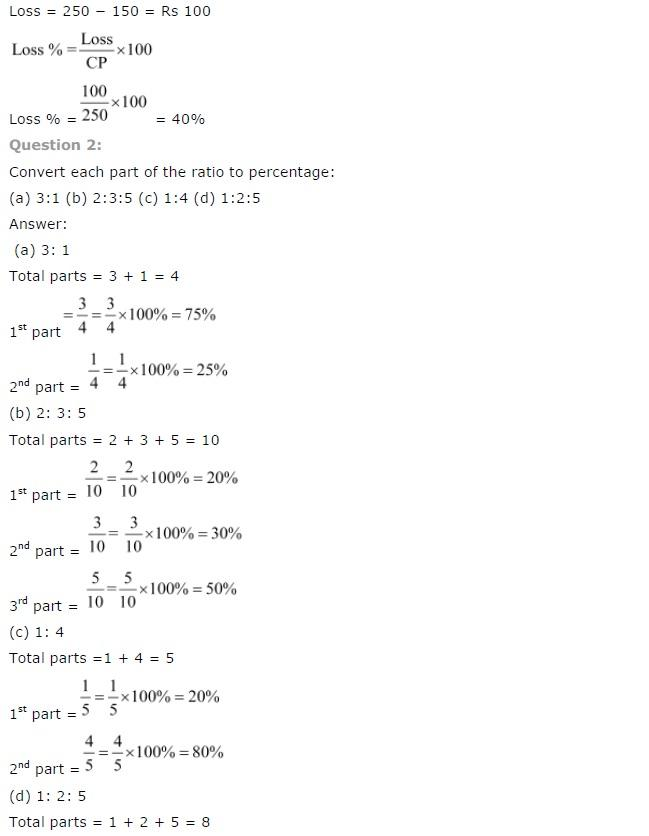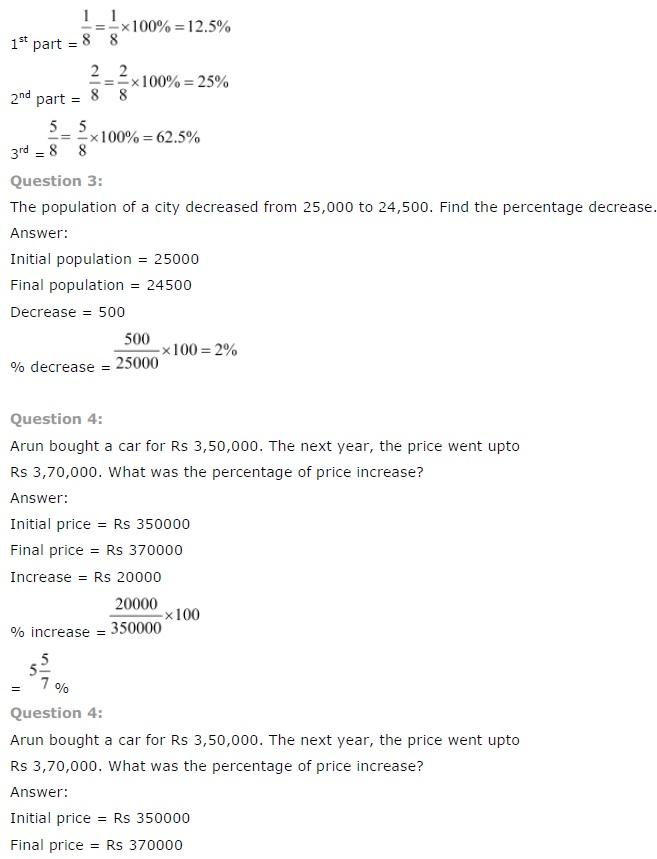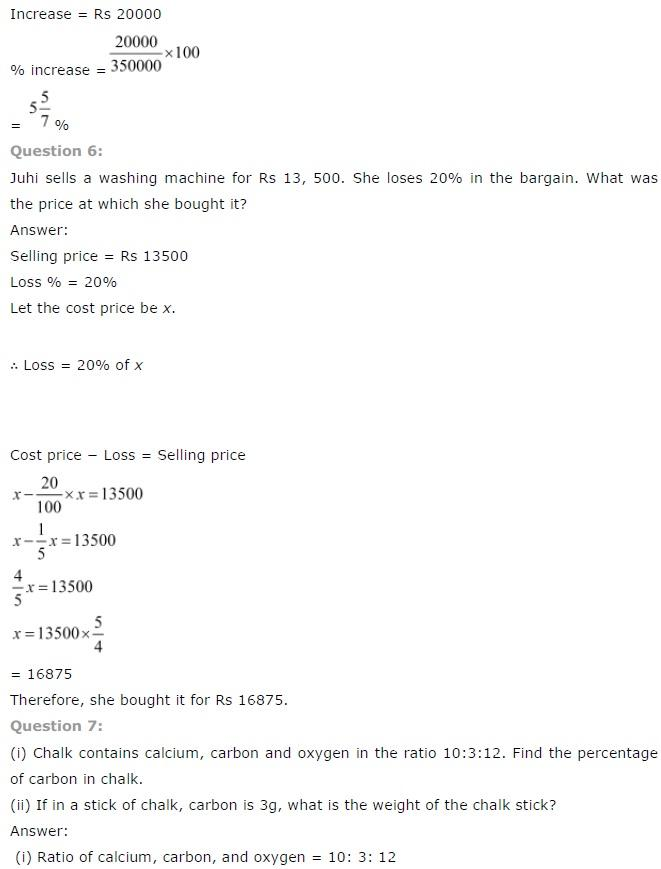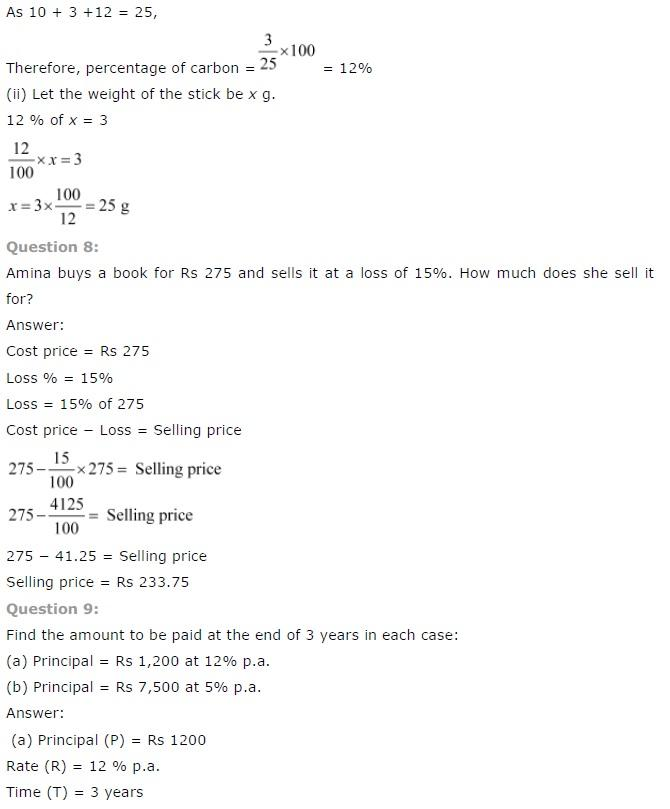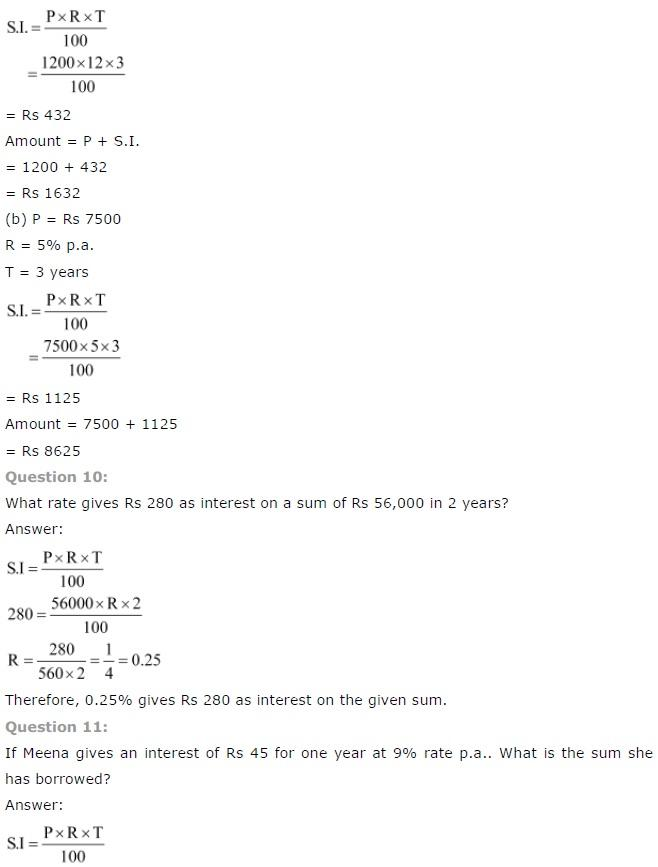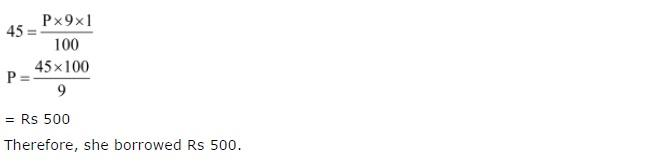NCERT CLASS 7 MATHEMATICS SOLUTIONS